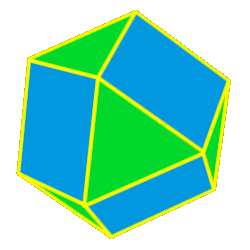# Polyhedra Cuboctahedron

 The CuboctahedronThe cuboctahedron is created by either truncating (cutting off) thecube one half of the way into each edge or by truncating the octahedron one half of the way into each side. 14 total faces: 8 equilateral triangles and 6 squares 12 vertices: 2 triangles and 2 squares 24 edges Dihedral angles:125 degrees, 16 minutes